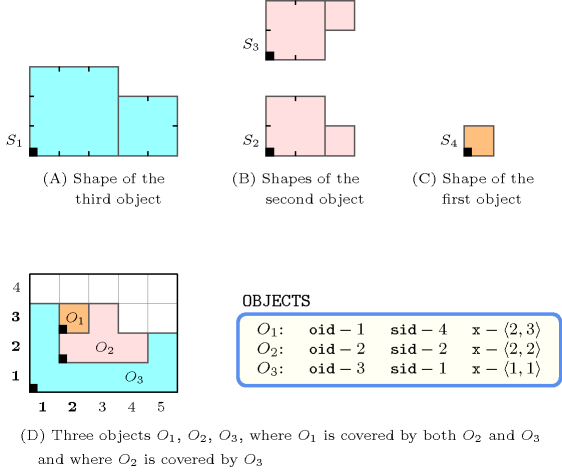## 5.93. coveredby_sboxes

Origin

Geometry, derived from [RandellCuiCohn92]

Constraint

$\mathrm{𝚌𝚘𝚟𝚎𝚛𝚎𝚍𝚋𝚢}_\mathrm{𝚜𝚋𝚘𝚡𝚎𝚜}\left(𝙺,\mathrm{𝙳𝙸𝙼𝚂},\mathrm{𝙾𝙱𝙹𝙴𝙲𝚃𝚂},\mathrm{𝚂𝙱𝙾𝚇𝙴𝚂}\right)$

Synonym

$\mathrm{𝚌𝚘𝚟𝚎𝚛𝚎𝚍𝚋𝚢}$.

Types
 $\mathrm{𝚅𝙰𝚁𝙸𝙰𝙱𝙻𝙴𝚂}$ $\mathrm{𝚌𝚘𝚕𝚕𝚎𝚌𝚝𝚒𝚘𝚗}\left(𝚟-\mathrm{𝚍𝚟𝚊𝚛}\right)$ $\mathrm{𝙸𝙽𝚃𝙴𝙶𝙴𝚁𝚂}$ $\mathrm{𝚌𝚘𝚕𝚕𝚎𝚌𝚝𝚒𝚘𝚗}\left(𝚟-\mathrm{𝚒𝚗𝚝}\right)$ $\mathrm{𝙿𝙾𝚂𝙸𝚃𝙸𝚅𝙴𝚂}$ $\mathrm{𝚌𝚘𝚕𝚕𝚎𝚌𝚝𝚒𝚘𝚗}\left(𝚟-\mathrm{𝚒𝚗𝚝}\right)$
Arguments
 $𝙺$ $\mathrm{𝚒𝚗𝚝}$ $\mathrm{𝙳𝙸𝙼𝚂}$ $\mathrm{𝚜𝚒𝚗𝚝}$ $\mathrm{𝙾𝙱𝙹𝙴𝙲𝚃𝚂}$ $\mathrm{𝚌𝚘𝚕𝚕𝚎𝚌𝚝𝚒𝚘𝚗}\left(\mathrm{𝚘𝚒𝚍}-\mathrm{𝚒𝚗𝚝},\mathrm{𝚜𝚒𝚍}-\mathrm{𝚍𝚟𝚊𝚛},𝚡-\mathrm{𝚅𝙰𝚁𝙸𝙰𝙱𝙻𝙴𝚂}\right)$ $\mathrm{𝚂𝙱𝙾𝚇𝙴𝚂}$ $\mathrm{𝚌𝚘𝚕𝚕𝚎𝚌𝚝𝚒𝚘𝚗}\left(\mathrm{𝚜𝚒𝚍}-\mathrm{𝚒𝚗𝚝},𝚝-\mathrm{𝙸𝙽𝚃𝙴𝙶𝙴𝚁𝚂},𝚕-\mathrm{𝙿𝙾𝚂𝙸𝚃𝙸𝚅𝙴𝚂}\right)$
Restrictions
 $|\mathrm{𝚅𝙰𝚁𝙸𝙰𝙱𝙻𝙴𝚂}|\ge 1$ $|\mathrm{𝙸𝙽𝚃𝙴𝙶𝙴𝚁𝚂}|\ge 1$ $|\mathrm{𝙿𝙾𝚂𝙸𝚃𝙸𝚅𝙴𝚂}|\ge 1$ $\mathrm{𝚛𝚎𝚚𝚞𝚒𝚛𝚎𝚍}$$\left(\mathrm{𝚅𝙰𝚁𝙸𝙰𝙱𝙻𝙴𝚂},𝚟\right)$ $|\mathrm{𝚅𝙰𝚁𝙸𝙰𝙱𝙻𝙴𝚂}|=𝙺$ $\mathrm{𝚛𝚎𝚚𝚞𝚒𝚛𝚎𝚍}$$\left(\mathrm{𝙸𝙽𝚃𝙴𝙶𝙴𝚁𝚂},𝚟\right)$ $|\mathrm{𝙸𝙽𝚃𝙴𝙶𝙴𝚁𝚂}|=𝙺$ $\mathrm{𝚛𝚎𝚚𝚞𝚒𝚛𝚎𝚍}$$\left(\mathrm{𝙿𝙾𝚂𝙸𝚃𝙸𝚅𝙴𝚂},𝚟\right)$ $|\mathrm{𝙿𝙾𝚂𝙸𝚃𝙸𝚅𝙴𝚂}|=𝙺$ $\mathrm{𝙿𝙾𝚂𝙸𝚃𝙸𝚅𝙴𝚂}.𝚟>0$ $𝙺>0$ $\mathrm{𝙳𝙸𝙼𝚂}\ge 0$ $\mathrm{𝙳𝙸𝙼𝚂}<𝙺$ $\mathrm{𝚒𝚗𝚌𝚛𝚎𝚊𝚜𝚒𝚗𝚐}_\mathrm{𝚜𝚎𝚚}$$\left(\mathrm{𝙾𝙱𝙹𝙴𝙲𝚃𝚂},\left[\mathrm{𝚘𝚒𝚍}\right]\right)$ $\mathrm{𝚛𝚎𝚚𝚞𝚒𝚛𝚎𝚍}$$\left(\mathrm{𝙾𝙱𝙹𝙴𝙲𝚃𝚂},\left[\mathrm{𝚘𝚒𝚍},\mathrm{𝚜𝚒𝚍},𝚡\right]\right)$ $\mathrm{𝙾𝙱𝙹𝙴𝙲𝚃𝚂}.\mathrm{𝚘𝚒𝚍}\ge 1$ $\mathrm{𝙾𝙱𝙹𝙴𝙲𝚃𝚂}.\mathrm{𝚘𝚒𝚍}\le |\mathrm{𝙾𝙱𝙹𝙴𝙲𝚃𝚂}|$ $\mathrm{𝙾𝙱𝙹𝙴𝙲𝚃𝚂}.\mathrm{𝚜𝚒𝚍}\ge 1$ $\mathrm{𝙾𝙱𝙹𝙴𝙲𝚃𝚂}.\mathrm{𝚜𝚒𝚍}\le |\mathrm{𝚂𝙱𝙾𝚇𝙴𝚂}|$ $\mathrm{𝚛𝚎𝚚𝚞𝚒𝚛𝚎𝚍}$$\left(\mathrm{𝚂𝙱𝙾𝚇𝙴𝚂},\left[\mathrm{𝚜𝚒𝚍},𝚝,𝚕\right]\right)$ $|\mathrm{𝚂𝙱𝙾𝚇𝙴𝚂}|\ge 1$ $\mathrm{𝚂𝙱𝙾𝚇𝙴𝚂}.\mathrm{𝚜𝚒𝚍}\ge 1$ $\mathrm{𝚂𝙱𝙾𝚇𝙴𝚂}.\mathrm{𝚜𝚒𝚍}\le |\mathrm{𝚂𝙱𝙾𝚇𝙴𝚂}|$ $\mathrm{𝚍𝚘}_\mathrm{𝚗𝚘𝚝}_\mathrm{𝚘𝚟𝚎𝚛𝚕𝚊𝚙}\left(\mathrm{𝚂𝙱𝙾𝚇𝙴𝚂}\right)$
Purpose

Holds if, for each pair of objects $\left({O}_{i},{O}_{j}\right)$, $i, ${O}_{i}$ is covered by ${O}_{j}$ with respect to a set of dimensions depicted by $\mathrm{𝙳𝙸𝙼𝚂}$. ${O}_{i}$ and ${O}_{j}$ are objects that take a shape among a set of shapes. Each shape is defined as a finite set of shifted boxes, where each shifted box is described by a box in a $𝙺$-dimensional space at a given offset (from the origin of the shape) with given sizes. More precisely, a shifted box is an entity defined by its shape id $\mathrm{𝚜𝚒𝚍}$, shift offset $𝚝$, and sizes $𝚕$. Then, a shape is defined as the union of shifted boxes sharing the same shape id. An object is an entity defined by its unique object identifier $\mathrm{𝚘𝚒𝚍}$, shape id $\mathrm{𝚜𝚒𝚍}$ and origin $𝚡$.

An object ${O}_{i}$ is covered by an object ${O}_{j}$ with respect to a set of dimensions depicted by $\mathrm{𝙳𝙸𝙼𝚂}$ if and only if, for all shifted box ${s}_{i}$ of ${O}_{i}$, there exists a shifted box ${s}_{j}$ of ${O}_{j}$ such that:

• For all dimensions $d\in \mathrm{𝙳𝙸𝙼𝚂}$, (1) the start of ${s}_{j}$ in dimension $d$ is less than or equal to the start of ${s}_{i}$ in dimension $d$, and (2) the end of ${s}_{i}$ in dimension $d$ is less than or equal to the end of ${s}_{j}$ in dimension $d$.

• There exists a dimension $d$ where, (1) the start of ${s}_{j}$ in dimension $d$ coincide with the start of ${s}_{i}$ in dimension $d$, or (2) the end of ${s}_{j}$ in dimension $d$ coincide with the end of ${s}_{i}$ in dimension $d$.

Example
$\left(\begin{array}{c}2,\left\{0,1\right\},\hfill \\ 〈\begin{array}{ccc}\mathrm{𝚘𝚒𝚍}-1\hfill & \mathrm{𝚜𝚒𝚍}-4\hfill & 𝚡-〈2,3〉,\hfill \\ \mathrm{𝚘𝚒𝚍}-2\hfill & \mathrm{𝚜𝚒𝚍}-2\hfill & 𝚡-〈2,2〉,\hfill \\ \mathrm{𝚘𝚒𝚍}-3\hfill & \mathrm{𝚜𝚒𝚍}-1\hfill & 𝚡-〈1,1〉\hfill \end{array}〉,\hfill \\ 〈\begin{array}{ccc}\mathrm{𝚜𝚒𝚍}-1\hfill & 𝚝-〈0,0〉\hfill & 𝚕-〈3,3〉,\hfill \\ \mathrm{𝚜𝚒𝚍}-1\hfill & 𝚝-〈3,0〉\hfill & 𝚕-〈2,2〉,\hfill \\ \mathrm{𝚜𝚒𝚍}-2\hfill & 𝚝-〈0,0〉\hfill & 𝚕-〈2,2〉,\hfill \\ \mathrm{𝚜𝚒𝚍}-2\hfill & 𝚝-〈2,0〉\hfill & 𝚕-〈1,1〉,\hfill \\ \mathrm{𝚜𝚒𝚍}-3\hfill & 𝚝-〈0,0〉\hfill & 𝚕-〈2,2〉,\hfill \\ \mathrm{𝚜𝚒𝚍}-3\hfill & 𝚝-〈2,1〉\hfill & 𝚕-〈1,1〉,\hfill \\ \mathrm{𝚜𝚒𝚍}-4\hfill & 𝚝-〈0,0〉\hfill & 𝚕-〈1,1〉\hfill \end{array}〉\hfill \end{array}\right)$

Figure 5.93.1 shows the objects of the example. Since ${O}_{1}$ is covered by both ${O}_{2}$ and ${O}_{3}$, and since ${O}_{2}$ is covered by ${O}_{3}$, the $\mathrm{𝚌𝚘𝚟𝚎𝚛𝚎𝚍𝚋𝚢}_\mathrm{𝚜𝚋𝚘𝚡𝚎𝚜}$ constraint holds.

##### Figure 5.93.1. (D) the three objects ${O}_{1}$, ${O}_{2}$, ${O}_{3}$ of the Example slot respectively assigned shapes ${S}_{4}$, ${S}_{2}$, ${S}_{1}$; (A), (B), (C) shapes ${S}_{1}$, ${S}_{2}$, ${S}_{3}$ and ${S}_{4}$ are respectively made up from 2, 2, 2 and 1 single shifted box.Typical
$|\mathrm{𝙾𝙱𝙹𝙴𝙲𝚃𝚂}|>1$
Symmetries
• Items of $\mathrm{𝚂𝙱𝙾𝚇𝙴𝚂}$ are permutable.

• Items of $\mathrm{𝙾𝙱𝙹𝙴𝙲𝚃𝚂}.𝚡$, $\mathrm{𝚂𝙱𝙾𝚇𝙴𝚂}.𝚝$ and $\mathrm{𝚂𝙱𝙾𝚇𝙴𝚂}.𝚕$ are permutable (same permutation used).

Remark

One of the eight relations of the Region Connection Calculus [RandellCuiCohn92]. The constraint $\mathrm{𝚌𝚘𝚟𝚎𝚛𝚎𝚍𝚋𝚢}_\mathrm{𝚜𝚋𝚘𝚡𝚎𝚜}$ is a restriction of the original relation since it requires that each shifted box of an object is covered by one shifted box of the other object.

 $•\mathrm{𝚘𝚛𝚒𝚐𝚒𝚗}\left(𝙾\mathtt{1},𝚂\mathtt{1},𝙳\right)\stackrel{\mathrm{def}}{=}𝙾\mathtt{1}.𝚡\left(𝙳\right)+𝚂\mathtt{1}.𝚝\left(𝙳\right)$ $•\mathrm{𝚎𝚗𝚍}\left(𝙾\mathtt{1},𝚂\mathtt{1},𝙳\right)\stackrel{\mathrm{def}}{=}𝙾\mathtt{1}.𝚡\left(𝙳\right)+𝚂\mathtt{1}.𝚝\left(𝙳\right)+𝚂\mathtt{1}.𝚕\left(𝙳\right)$ $•\begin{array}{c}\mathrm{𝚌𝚘𝚟𝚎𝚛𝚎𝚍𝚋𝚢}_\mathrm{𝚜𝚋𝚘𝚡𝚎𝚜}\left(\mathrm{𝙳𝚒𝚖𝚜},𝙾\mathtt{1},𝚂\mathtt{1},𝙾\mathtt{2},𝚂\mathtt{2}\right)\stackrel{\mathrm{def}}{=}\hfill \\ \bigwedge \left(\begin{array}{c}\begin{array}{c}\forall 𝙳\in \mathrm{𝙳𝚒𝚖𝚜}\hfill \\ \bigwedge \left(\begin{array}{c}\begin{array}{c}\mathrm{𝚘𝚛𝚒𝚐𝚒𝚗}\left(\begin{array}{c}𝙾\mathtt{2},\hfill \\ 𝚂\mathtt{2},\hfill \\ 𝙳\hfill \end{array}\right)\le \hfill \\ \mathrm{𝚘𝚛𝚒𝚐𝚒𝚗}\left(\begin{array}{c}𝙾\mathtt{1},\hfill \\ 𝚂\mathtt{1},\hfill \\ 𝙳\hfill \end{array}\right)\hfill \end{array},\hfill \\ \begin{array}{c}\mathrm{𝚎𝚗𝚍}\left(𝙾\mathtt{1},𝚂\mathtt{1},𝙳\right)\le \hfill \\ \mathrm{𝚎𝚗𝚍}\left(𝙾\mathtt{2},𝚂\mathtt{2},𝙳\right)\hfill \end{array}\hfill \end{array}\right)\hfill \end{array},\hfill \\ \begin{array}{c}\exists 𝙳\in \mathrm{𝙳𝚒𝚖𝚜}\hfill \\ \bigvee \left(\begin{array}{c}\begin{array}{c}\mathrm{𝚘𝚛𝚒𝚐𝚒𝚗}\left(\begin{array}{c}𝙾\mathtt{2},\hfill \\ 𝚂\mathtt{2},\hfill \\ 𝙳\hfill \end{array}\right)=\hfill \\ \mathrm{𝚘𝚛𝚒𝚐𝚒𝚗}\left(\begin{array}{c}𝙾\mathtt{1},\hfill \\ 𝚂\mathtt{1},\hfill \\ 𝙳\hfill \end{array}\right)\hfill \end{array},\hfill \\ \begin{array}{c}\mathrm{𝚎𝚗𝚍}\left(𝙾\mathtt{1},𝚂\mathtt{1},𝙳\right)=\hfill \\ \mathrm{𝚎𝚗𝚍}\left(𝙾\mathtt{2},𝚂\mathtt{2},𝙳\right)\hfill \end{array}\hfill \end{array}\right)\hfill \end{array}\hfill \end{array}\right)\hfill \end{array}$ $•\begin{array}{c}\mathrm{𝚌𝚘𝚟𝚎𝚛𝚎𝚍𝚋𝚢}_\mathrm{𝚘𝚋𝚓𝚎𝚌𝚝𝚜}\left(\mathrm{𝙳𝚒𝚖𝚜},𝙾\mathtt{1},𝙾\mathtt{2}\right)\stackrel{\mathrm{def}}{=}\hfill \\ \begin{array}{c}\forall 𝚂\mathtt{1}\in \mathrm{𝚜𝚋𝚘𝚡𝚎𝚜}\left(\left[𝙾\mathtt{1}.\mathrm{𝚜𝚒𝚍}\right]\right)\hfill \\ \begin{array}{c}\exists 𝚂\mathtt{2}\in \mathrm{𝚜𝚋𝚘𝚡𝚎𝚜}\left(\begin{array}{c}\left[\begin{array}{c}𝙾\mathtt{2}.\mathrm{𝚜𝚒𝚍}\hfill \end{array}\right]\hfill \end{array}\right)\hfill \\ \mathrm{𝚌𝚘𝚟𝚎𝚛𝚎𝚍𝚋𝚢}_\mathrm{𝚜𝚋𝚘𝚡𝚎𝚜}\left(\begin{array}{c}\mathrm{𝙳𝚒𝚖𝚜},\hfill \\ 𝙾\mathtt{1},\hfill \\ 𝚂\mathtt{1},\hfill \\ 𝙾\mathtt{2},\hfill \\ 𝚂\mathtt{2}\hfill \end{array}\right)\hfill \end{array}\hfill \end{array}\hfill \end{array}$ $•\begin{array}{c}\mathrm{𝚊𝚕𝚕}_\mathrm{𝚌𝚘𝚟𝚎𝚛𝚎𝚍𝚋𝚢}\left(\mathrm{𝙳𝚒𝚖𝚜},\mathrm{𝙾𝙸𝙳𝚂}\right)\stackrel{\mathrm{def}}{=}\hfill \\ \begin{array}{c}\forall 𝙾\mathtt{1}\in \mathrm{𝚘𝚋𝚓𝚎𝚌𝚝𝚜}\left(\mathrm{𝙾𝙸𝙳𝚂}\right)\hfill \\ \begin{array}{c}\forall 𝙾\mathtt{2}\in \mathrm{𝚘𝚋𝚓𝚎𝚌𝚝𝚜}\left(\mathrm{𝙾𝙸𝙳𝚂}\right)\hfill \\ \begin{array}{c}\begin{array}{c}𝙾\mathtt{1}.\mathrm{𝚘𝚒𝚍}<\hfill \\ 𝙾\mathtt{2}.\mathrm{𝚘𝚒𝚍}\hfill \end{array}⇒\hfill \\ \mathrm{𝚌𝚘𝚟𝚎𝚛𝚎𝚍𝚋𝚢}_\mathrm{𝚘𝚋𝚓𝚎𝚌𝚝𝚜}\left(\begin{array}{c}\mathrm{𝙳𝚒𝚖𝚜},\hfill \\ 𝙾\mathtt{1},\hfill \\ 𝙾\mathtt{2}\hfill \end{array}\right)\hfill \end{array}\hfill \end{array}\hfill \end{array}\hfill \end{array}$ $•\mathrm{𝚊𝚕𝚕}_\mathrm{𝚌𝚘𝚟𝚎𝚛𝚎𝚍𝚋𝚢}\left(\mathrm{𝙳𝙸𝙼𝙴𝙽𝚂𝙸𝙾𝙽𝚂},\mathrm{𝙾𝙸𝙳𝚂}\right)$